# Geometric progression + exponential function - math problems

#### Number of problems found: 36

• Geometric progressionIn geometric progression, a1 = 7, q = 5. Find the condition for n to sum first n members is: sn≤217.
• SequenceCalculate what member of the sequence specified by ? has value 86.
• Sequence - 5 membersWrite first five members of the sequence ?
• PopulationWhat is the population of the city with 3% annual growth, if in 10 years the city will have 60,000 residents?
• LoanApply for a \$ 59000 loan, the loan repayment period is 8 years, the interest rate 7%. How much should I pay for every month (or every year if paid yearly). Example is for practise geometric progression and/or periodic payment for an annuity.A radioactive material loses 10% of its mass each year. What proportion will be left there after n=6 years?
• DemographicsThe population grew in the city in 10 years from 30000 to 34000. What is the average annual percentage increase of population?
• Investment1000\$ is invested at 10% compound interest. What factor is the capital multiplied by each year? How much will be there after n=12 years?
• InterestCalculate how much you earn for 10 years 43000 deposit if the interest rate is 1.3% and the interest period is a quarter.
• DepositIf you deposit 719 euros the beginning of each year, how much money we have at 1.3% (compound) interest after 9 years?
• Half life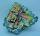Determine the half life of bismuth, when bismuth weight from the original weight of 32 g was only 2 grams in 242 minutes.
• PopulationThe town has 65,000 inhabitants. 40 years ago, there were 157,000. How many people will live in a city in 10 years if the population's average rate is as in previous years?
• The city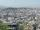At the end of 2010 the city had 248000 residents. The population increased by 2.5% each year. What is the population at the end of 2013?
• Annual interest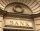A loan of 10 000 euro is to be repaid in annual payments over 10 years. Assuming a fixed 10% annual interest rate compounded annually, calculate: (a) the amount of each annual repayment (b) the total interest paid.
• Annual growthThe population has grown from 25,000 to 33,600 in 10 years. Calculate what was the average annual population growth in%?
• Common ratio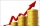If 200 units of a commodity are consumed in a first year, and if the annual rate of increase in consumption is 5% (a) what amount is consumed in the 8th year; (b) in the first 15 years?
• CrystalThe crystal grows every month 1.2 permille of its mass. For how many months to grow a crystal from weight 177 g to 384 g?
• The crime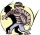The crime rate of a certain city is increasing by exactly 7% each year. If there were 600 crimes in the year 1990 and the crime rate remains constant each year, determine the approximate number of crimes in the year 2025.
• Microorganisms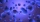The first generation of micro-organisms has a population of 13500 members. Each next generation is 11/10 times the previous one. Find out how many generations will reach at least three times members of the first generation.
• BankPaul put 10000 in the bank for 6 years. Calculate how much you will have in the bank if he not pick earned interest or change deposit conditions. The annual interest rate is 3.5%, and the tax on interest is 10%.

Do you have an interesting mathematical word problem that you can't solve it? Submit a math problem, and we can try to solve it.

We will send a solution to your e-mail address. Solved examples are also published here. Please enter the e-mail correctly and check whether you don't have a full mailbox.

Please do not submit problems from current active competitions such as Mathematical Olympiad, correspondence seminars etc...

Geometric progression - math word problems. Exponential function - math word problems.